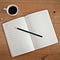NLP & Deep learning | Always a learner

# Time and Space Complexity of Machine Learning Models

Time complexity is an essential aspect to know when anyone wants their model with low latency. Let’s dive deep into details of how much time and space required by the wide variety of models to predict the output.

“Assuming training data has n points with d dimensions “

# 1) K-Nearest Neighbors:

Given query point (xq), K-NN follows these steps to predict output (yq). …

# 1) Contents

a. Data Collection

b. Exploratory Data Analysis

c. Data Preprocessing

d. feature engineering

e. Feature Selection

f. Model Selection and Hyperparameter Tuning

h. Model Evaluation and Analysis

# Introduction:

It's very important to know where our model works well and where it fails. If there is a low latency requirement, definitely KNN will be a worse choice. Similarly, if data is non-linear, then choosing logistic regression is not good so let's dive deep into the discussion and find the pros and cons of models.

# Hypothesis test with an example

Untangle hypothesis testing with a detailed walkthrough

# Example:

Consider C1, C2 population heights of students fom two classooms. The problem is to prove that the mean heights of C1 and C2 are the same.

# Steps to follow for Hypothesis Testing:

## a)Choose the Test Statistic:

Observed difference in mean (uc1-uc2) = 30

# Introduction:

This blog strictly limits to code walkthrough to generate a summary using Text to text transfer transformer(T-5). If you guys are curious about how T-5 works and how it was pretrained and fine-tuned on downstream NLP tasks check out the following the blog.

# 5 Great Deep learning projects for beginnersPhoto by Hope House Press — Leather Diary Studio on Unsplash

Deep learning a trending word in technology for the past 6 years and hundreds of research papers have been publishing every week with new techniques to solve various Natural Language Processing, Natural Language Understanding, and computer vision tasks.

However, as a beginner, one has to be focus on the basics and need to understand how things work.

# Introduction:

These are some interesting questions I encountered while preparing for a machine learning interview and tried to answer them.

Check out this for the first part